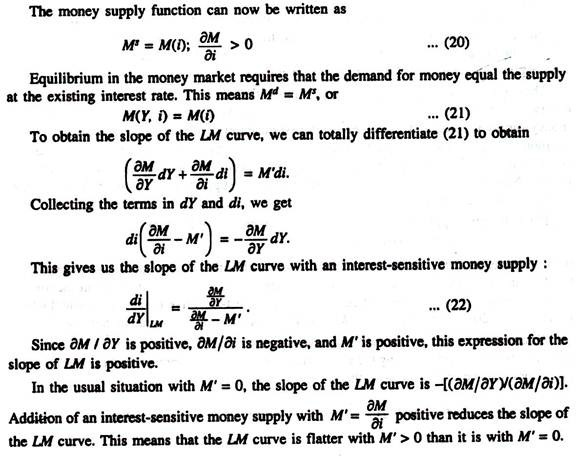# Effect of Money Supply on the LM-Curve (With Diagram) | Supply of Money

Let us make an in-depth study of Money Supply on the LM-Curve.

The interest sensitivity of the money supply can now be shown in terms of the IS-LM curve model.

Fig. 20.5 shows the supply and demand curves for money balances M.

With an initial income level Y0, the demand-for-money curve is M(Y0).

With an initial money supply M0 we hold P constant throughout this analysis. So we do not draw a distinction between nominal balance and real balance. The interest rate is r0. Now suppose income rises, due to a rightward shift of the IS curve. Then the demand curve in Fig 20.5 shifts up to M(Y1), and with a money supply fixed at M0, the interest rate will rise to i1.

But if the money supply is responsive to interest rate changes, the money supply function through the initial equilibrium point i0, M0 (Y0) will be the positively sloped supply function M(i – id) in Fig. 20.5. In this case, the increased demand for money leads to an increase in supply equal to M1 – M0 as the interest rate rises to i2 instead of i1.

Thus, the interest elasticity of the money supply reduces the increase in i (from i1 – i0 to i2 – i0) needed to maintain money market equilibrium with a given increase in Y, from Y0 to Y1, in Fig. 20.5.

Thus with an interest-sensitive money supply, the slope of the LM curve is flatter than otherwise. This is shown in LM diagram of Fig. 20.6. At the initial interest rate i0, the real money supply is equal to M(i0) in the southwest quadrant. If the interest rate rises to i1, with the money supply fixed at M(i0), the level of income must rise to Y1 to maintain money market equilibrium.

The interest rate increase reduces the speculative and transactions demand for money. Now it is not necessary for money supply to support an increase in Y within the constraint of a given M.

If the money supply is not fixed, but is a function of the interest rate i: